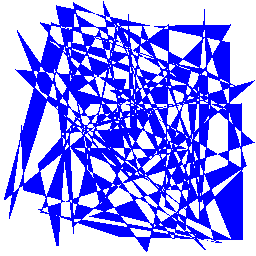## Saturday, October 09, 2010

### Is Pygame faster than PIL? How about pyglet?

In my work I draw a lot of polygons on PNG images.Currently I use Python Image Library (PIL) for all my drawing and I'm pretty happy with the performance. Intriguingly, I read here that "Pygame is three or four times faster than PIL" and that got me interested.

I guess it makes sense that a library designed for games would be better optimised than alternatives. I created this simple test to render a random polygon and measure the speed.

`import timeimport randomimport osfrom PIL import Image, ImageDrawimport pygamedef PolygonPILTester(polygon):    width = 256    height = 256    img = Image.new("RGBA", (width, height), (255, 255, 255, 0)) # rgba    draw = ImageDraw.Draw(img)    draw.polygon(polygon, fill="blue")    f = open("pil.png", "wb")    img.save(f, "PNG")    f.close()def PolygonPyGameTester(polygon):    width = 256    height = 256    os.environ['SDL_VIDEODRIVER'] = 'dummy'    pygame.display.init()    pygame.display.set_mode((1,1), 0, 32)    surf = pygame.Surface((256, 256), pygame.SRCALPHA)    pygame.draw.polygon(surf, pygame.Color("blue"), polygon)    pygame.image.save(surf, 'pygame.png')if __name__ == "__main__":    polygon = []    for i in range(100):    polygon.append((random.randint(1,255), random.randint(1,255)))        start = time.time()    PolygonPILTester(polygon)    end = time.time()    print("PIL took %s seconds" % (end - start))    start = time.time()    PolygonPyGameTester(polygon)    end = time.time()    print("pygame took %s seconds" % (end - start))`

Here's pygame's version:Interestingly in this test, PIL is twice as fast as pygame, here's a typical result:

PIL took 0.015594959259 seconds

pygame took 0.0300869941711 seconds

My guess is that as the author of the gheat code found the reverse that pygame is better at image compositing which is used more for games than for just drawing polygons.

There's another python image library out there, pyglet, but so far I haven't figured out how to do a simple drawing on a new image and save to a PNG file without it wanting an X server. I get "pyglet.window.NoSuchDisplayException: Cannot connect to ""." Any pointers would be appreciated..